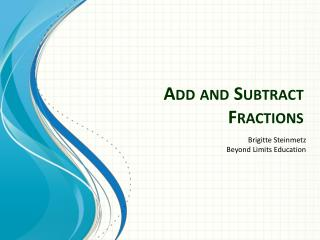DownloadDownload PresentationDownload Presentation- - - - - - - - - - - - - - - - - - - - - - - - - - - E N D - - - - - - - - - - - - - - - - - - - - - - - - - - -
##### Presentation Transcript

1. Add and SubtractFractions Brigitte Steinmetz Beyond Limits Education

2. Objectives • To add/subtract fractions that have common denominators. • To add/subtract fractions that have uncommon denominators using the least common denominator. • To estimate sums and differences with fractions using benchmarks. • To identify what information should be written down in Cornell notes.

3. Add Like Fractions • Using your fraction cubes, make the following fractions: 38 58 + Now add them together. 1/8 1/8 1/8 1/8 1/8 1/8 1/8 1/8

4. Add Like Fractions 38 58 + 88 1/8 = 1/8 1 1/8 1 1 = 1/8 1/8 1/8 1/8 1/8 1/8

5. Subtract Like Fractions • Using your fraction cubes, make the following fraction: 58 38 - Now subtract 3 eights. 1/8 1/8 1/8 1/8 1/8

6. Subtract Like Fractions 58 38 - 28 1/8 = 1/8 = 14 1/8 1 4 1/8 1/8

7. Add Unlike Fractions • Using your fraction cubes, make the following fractions: 12 14 + Now add them together. + 12 14

8. Add Unlike Fractions 14 14 14 14 12 24 12 = 24 14 + 34

9. Subtract Unlike Fractions • Using your fraction cubes, make the following fraction: 23 12 - Now subtract 1 half. 16 13 16 - 12 16 13 16

10. Subtract Unlike Fractions = ? 16 16 13 16 12 16 13 16

11. Estimate Sums & Differences • Using your fraction cubes number line, estimate the sum of: 38 45 + 1/8 4/8 5/8 6/8 7/8 8/8 2/8 3/8 1/2 0 1 1 2 38 close to

12. Estimate Sums & Differences • Using your fraction cubes number line, estimate the sum of: 38 45 + 2/5 3/5 1/5 4/5 1/2 0 1 45 close to 1

13. Estimate Sums & Differences • Using your fraction cubes number line, estimate the sum of: 38 45 + 1 2 1 2 + = 1 1

14. Add/Subtract Unlike Fractions • To add/subtract unlike fractions you need to rename them as like fractions. • Least Common Denominator – is the least common multiple of two or more denominators.

15. Add Unlike Fractions • Using your fraction cubes, make the following fractions: 23 14 + Now add them together. 16 13 16 16 13 14 16

16. Add Unlike Fractions 23 Multiples of 3: 3, 6, 9, 12 Multiples of 4: 4, 8, 12 14 1/12 16 13 1/12 1/12 16 1/12 1/12 1/12 LCD = 12 16 13 1/12 1/12 14 1/12 1/12 1/12 1/12 16 1/12 1/12 1/12 1/12

17. Add Unlike Fractions 23 44 812 = X 14 33 312 = X

18. Add Unlike Fractions 812 312 + 1112

19. Subtract Unlike Fractions • Using your fraction cubes, make the following fraction: 34 16 - Now subtract 1 sixth. 14 14 14 16

20. Subtract Unlike Fractions 34 Multiples of 4: 4, 8, 12 Multiples of 6: 6, 12 1/12 16 14 1/12 1/12 1/12 14 1/12 1/12 LCD = 12 1/12 1/12 1/12 16 1/12 14 1/12 1/12 1/12 1/12 1/12 1/12

21. Subtract Unlike Fractions 34 33 912 = X 16 22 212 = X

22. Subtract Unlike Fractions 912 212 - 712

23. Add Mixed Fractions • Using your fraction cubes, make the following fractions: 3 8 14 2 + 1 Now add them together. 1 1 1 1/8 14 1/8 1/8

24. Add Mixed Fractions 3 8 2 Multiples of 4: 4, 8 Multiples of 8: 8 14 1 1 1 1 LCD = 8 1/8 14 1/8 1/8 1/8 1/8

25. Add Mixed Fractions 11 3 8 3 8 2 2 = X 22 2 8 14 1 1 = X

26. Add Mixed Fractions 3 8 2 2 8 1 + 5 8 3

27. Subtract Mixed Fractions • Using your fraction cubes, make the following fractions: 3 8 14 2 - 1 Now subtract one and one fourth. 1 1 1/8 1/8 1/8

28. Subtract Mixed Fractions 3 8 2 Multiples of 4: 4, 8 Multiples of 8: 8 14 1 1 1 1 LCD = 8 1/8 14 1/8 1/8 1/8 1/8

29. Subtract Mixed Fractions 11 3 8 3 8 2 2 = X 22 2 8 14 1 1 = X

30. Subtract Mixed Fractions 3 8 2 1 1 2 8 1 - 1 8 1 1/8 1/8 1/8

31. Subtract Mixed Fractions 3 8 2 2 8 1 - 1 8 1

32. Subtract Mixed Fractions • Using your fraction cubes, make the following fraction: 3 8 1 2 - Now subtract one and three eighths. 1 1

33. Subtract Mixed Fractions • In order to do this you must rename one of the two whole numbers. 8 8 1 2 = 1/8 1 1 1 1/8 1/8 1/8 1/8 1/8 1/8 1/8

34. Subtract Mixed Fractions • Now go through the same steps as before to… 3 8 1 2 - 8 8 1 1/8 1 1/8 3 8 1 - 1/8 1/8 1/8 5 8 1/8 1/8 1/8